# 1.7 – Variance and Standard Deviation

Previous: 1.6 – Expected Value

Next: 1.8 – Grade Distribution Example

Another important quantity related to a given random variable is its variance. The variance is a numerical description of the spread, or the dispersion, of the random variable. That is, the variance of a random variable X is a measure of how spread out the values of X are, given how likely each value is to be observed.

Definition: Variance and Standard Deviation of a Discrete Random Variable
The variance, Var(X), of a discrete random variable X is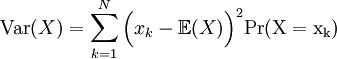The integer N is the number of possible values of X.

The standard deviation, σ, is the positive square root of the variance: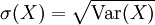Observe that the variance of a distribution is always non-negative (pk is non-negative, and the square of a number is also non-negative).

Observe also that much like the expectation of a random variable X, the variance (or standard deviation) is a weighted average of an expression of observable and calculable values. More precisely, notice that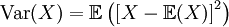Students in MATH 105 are expected to memorize the formulas for variance and standard deviation.

Using the grade distribution example of the previous page, calculate the variance and standard deviation of the random variable associated to randomly selecting a single exam.

## Solution

The variance of the random variable X is given by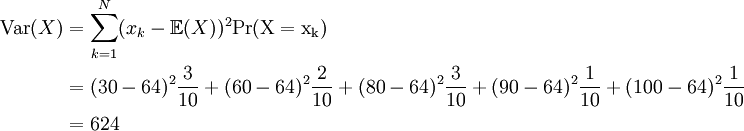The standard deviation of X is then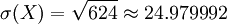## Interpretation of the Standard Deviation

For most "nice" random variables, i.e. ones that are not too wildly distributed, the standard deviation has a convenient informal interpretation. Consider the intervals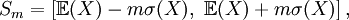for some positive integer m. As we increase the value of m, these intervals will contain more of the possible values of the random variable X.

A good rule of thumb is that for "nicely distributed" random variables, all of the most likely possible values of the random variable will be contained in the interval S3. Another way to say this is that most of the PDF will live on the interval S3.

For our grade distribution example, notice that all possible values of X are contained in the interval S3. In fact, all possible values of X are contained in S2 for this particular example.

Previous: 1.6 – Expected Value

Next: 1.8 – Grade Distribution Example